GATE  >  Test: Analog & Digital Electronics - 1

# Test: Analog & Digital Electronics - 1

Test Description

## 10 Questions MCQ Test GATE Electrical Engineering (EE) 2023 Mock Test Series | Test: Analog & Digital Electronics - 1

Test: Analog & Digital Electronics - 1 for GATE 2023 is part of GATE Electrical Engineering (EE) 2023 Mock Test Series preparation. The Test: Analog & Digital Electronics - 1 questions and answers have been prepared according to the GATE exam syllabus.The Test: Analog & Digital Electronics - 1 MCQs are made for GATE 2023 Exam. Find important definitions, questions, notes, meanings, examples, exercises, MCQs and online tests for Test: Analog & Digital Electronics - 1 below.
Solutions of Test: Analog & Digital Electronics - 1 questions in English are available as part of our GATE Electrical Engineering (EE) 2023 Mock Test Series for GATE & Test: Analog & Digital Electronics - 1 solutions in Hindi for GATE Electrical Engineering (EE) 2023 Mock Test Series course. Download more important topics, notes, lectures and mock test series for GATE Exam by signing up for free. Attempt Test: Analog & Digital Electronics - 1 | 10 questions in 30 minutes | Mock test for GATE preparation | Free important questions MCQ to study GATE Electrical Engineering (EE) 2023 Mock Test Series for GATE Exam | Download free PDF with solutions
 1 Crore+ students have signed up on EduRev. Have you?
Test: Analog & Digital Electronics - 1 - Question 1

### A voltage V = 4 sin ωt is applied to the terminals A and B of the circuit shown in figure. The diodes are assumed to be ideal. The impedance offered by the circuit across the terminals A and B in kilo-ohms is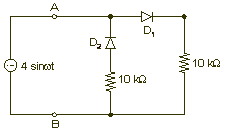Test: Analog & Digital Electronics - 1 - Question 2

### Consider the following limiter circuit an input voltage V| = 10.5 sinlOOnt is applied. Assume that the diode drop is 0.7 V in forward bias. The zener breakdown voltage is 6.3 V.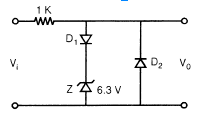The maximum and minimum values of the output voltage respectively are

Detailed Solution for Test: Analog & Digital Electronics - 1 - Question 2

For +ve value of Vi, D1 is F.B and zener diode regulates the voltage at 6.3 V across it.
V0 = 6.3 + 0.7 = 7 V
and for negative half of V:, D2 is forward biased.
'    V0 = -0.7 V

Test: Analog & Digital Electronics - 1 - Question 3

### In the saturation region of a BJT (Bipolar Junction Transistor)

Detailed Solution for Test: Analog & Digital Electronics - 1 - Question 3

Both junctions could be forward biased.

Test: Analog & Digital Electronics - 1 - Question 4

Given below is the Ic Vs VCE characteristic of two npn transistor P and Q having current gains β1 and β2 respectively.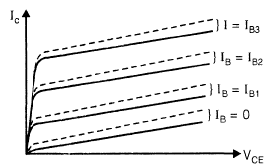Hard lines: Characteristics of P Dashed lines: Characteristics of Q

Which of the following is true?

Detailed Solution for Test: Analog & Digital Electronics - 1 - Question 4

β of Q should be >> β of P.

Test: Analog & Digital Electronics - 1 - Question 5

The thermal run-away in a CE-transistor amplifier can be prevented by biasing in such a wav that.

Detailed Solution for Test: Analog & Digital Electronics - 1 - Question 5

It is derived from the basic condition.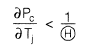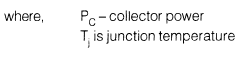Test: Analog & Digital Electronics - 1 - Question 6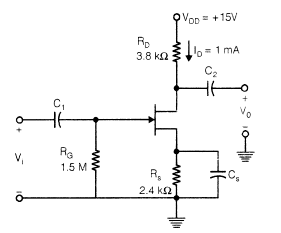IDSS = 8 mA Vp = -5 V, Assume Gate current » 0 all the capacitance used are of very large value. The voltage gain Av, is

Detailed Solution for Test: Analog & Digital Electronics - 1 - Question 6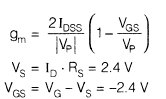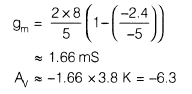Test: Analog & Digital Electronics - 1 - Question 7

If the differential voltage gain and the common mode voltage gain of a differential amplifier are 50-dB and 2-dB resDectivelv. then its common mode rejection ratio is

Detailed Solution for Test: Analog & Digital Electronics - 1 - Question 7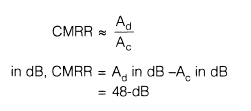Test: Analog & Digital Electronics - 1 - Question 8

Which of the following instruments has a uniform scale?

Detailed Solution for Test: Analog & Digital Electronics - 1 - Question 8

In the PMMC type instrument, the deflection is directly proportional to the current flowing through the instrument, we get a uniform scale for the instrument. It gives a uniform scale of upto 270° or more.

Test: Analog & Digital Electronics - 1 - Question 9

Consider the following op-amp circuit with multiple stages: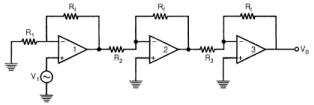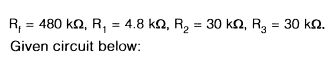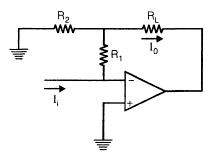Detailed Solution for Test: Analog & Digital Electronics - 1 - Question 9

I0 = kIi (current controlled current source).

Test: Analog & Digital Electronics - 1 - Question 10

Shown below is a positive feedback arrangement to generate sinusoidal oscillation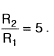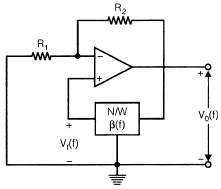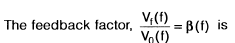Detailed Solution for Test: Analog & Digital Electronics - 1 - Question 10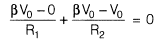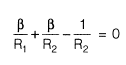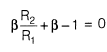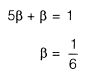## GATE Electrical Engineering (EE) 2023 Mock Test Series

22 docs|274 tests
Information about Test: Analog & Digital Electronics - 1 Page
In this test you can find the Exam questions for Test: Analog & Digital Electronics - 1 solved & explained in the simplest way possible. Besides giving Questions and answers for Test: Analog & Digital Electronics - 1, EduRev gives you an ample number of Online tests for practice

## GATE Electrical Engineering (EE) 2023 Mock Test Series

22 docs|274 tests(Scan QR code)